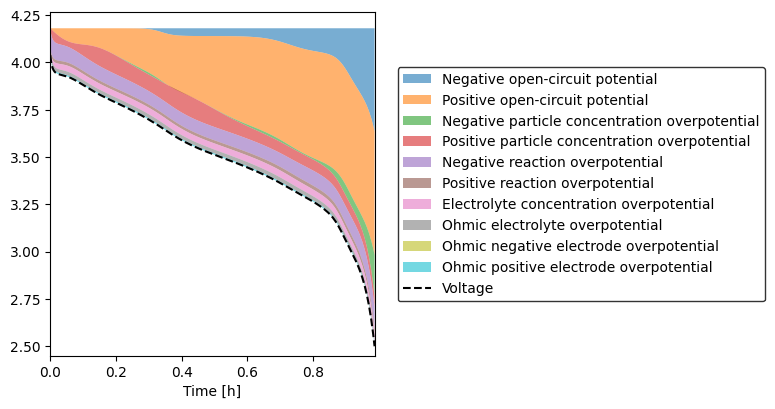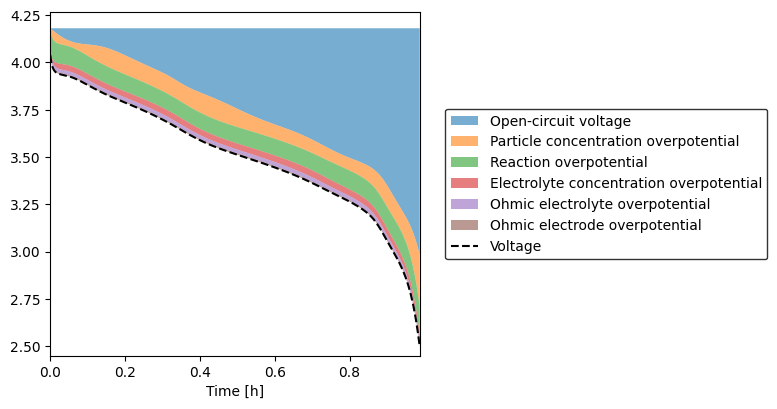Tip

An interactive online version of this notebook is available, which can be accessed via# Plot voltage components#

In a 1D model, the voltage of a cell is given by the difference in solid-phase potentials at the two edges of the domain

$V = \left.\phi_\mathrm{s,p}\right\rvert_{x=L} - \left.\phi_\mathrm{s,n}\right\rvert_{x=0}$

We can decompose this voltage into constituent components by adding and subtracting terms and regrouping:

\begin{split}\begin{align*} V =& \left(\left.\phi_\mathrm{s,p}\right\rvert_{x=L} - \bar{\phi}_\mathrm{s,p}\right) &\quad&\text{Positive solid phase ohmic losses} \\ &- \left(\left.\phi_\mathrm{s,n}\right\rvert_{x=L} - \bar{\phi}_\mathrm{s,n}\right) &&\text{Negative solid phase ohmic losses} \\ &+ \bar{\eta}_\mathrm{p} &&\text{Positive reaction overpotential} \\ &- \bar{\eta}_\mathrm{n} &&\text{Negative reaction overpotential} \\ &+ \left(\bar{\phi}_\mathrm{e,p}-\bar{\phi}_\mathrm{e,n}\right) &&\text{Electrolyte overpotential} \\ &+ \left(\overline{U(c_\mathrm{p}^\mathrm{surf})}-U(\bar{c}_\mathrm{p})\right) &&\text{Positive particle overpotential} \\ &- \left(\overline{U(c_\mathrm{n}^\mathrm{surf})}-U(\bar{c}_\mathrm{n})\right) &&\text{Negative particle overpotential} \\ &+ U(\bar{c}_\mathrm{p}) &&\text{Positive bulk open-circuit potential} \\ &- U(\bar{c}_\mathrm{n}) &&\text{Negative bulk open-circuit potential} \end{align*}\end{split}

where

$\eta = \phi_\mathrm{s} - \phi_\mathrm{e} - U(c^\mathrm{surf})$

and $$\bar{\cdot}$$ represents the average value of the variable over its subdomain.

To demonstrate this, we solve a standard DFN model with the Chen2020 parameter set and plot the various potentials

:

%pip install "pybamm[plot,cite]" -q    # install PyBaMM if it is not installed
import pybamm

model = pybamm.lithium_ion.DFN()

experiment = pybamm.Experiment(["Discharge at 1C until 2.5 V"])

sim = pybamm.Simulation(
model, experiment=experiment, parameter_values=pybamm.ParameterValues("Chen2020")
)
sol = sim.solve()

sol.plot(
[
"Negative electrode bulk open-circuit potential [V]",
"Positive electrode bulk open-circuit potential [V]",
"Negative particle concentration overpotential [V]",
"Positive particle concentration overpotential [V]",
"X-averaged negative electrode reaction overpotential [V]",
"X-averaged positive electrode reaction overpotential [V]",
"X-averaged concentration overpotential [V]",
"X-averaged electrolyte ohmic losses [V]",
"X-averaged negative electrode ohmic losses [V]",
"X-averaged positive electrode ohmic losses [V]",
],
)

Note: you may need to restart the kernel to use updated packages.

At t = 472.93 and h = 1.932e-14, the corrector convergence failed repeatedly or with |h| = hmin.
At t = 232.93 and h = 3.07648e-13, the corrector convergence failed repeatedly or with |h| = hmin.

:

<pybamm.plotting.quick_plot.QuickPlot at 0x1217ec4f0>


A more useful visualization is given by the plot_voltage_components function, which can either plot all the individual voltage components

:

pybamm.plot_voltage_components(sol, split_by_electrode=True):

(<Figure size 800x400 with 1 Axes>, <AxesSubplot: xlabel='Time [h]'>)


or group positive and negative together

:

pybamm.plot_voltage_components(sol):

(<Figure size 800x400 with 1 Axes>, <AxesSubplot: xlabel='Time [h]'>)


Note that this function further splits the electrolyte overpotential into the contribution from the electrolyte gradient and the contribution from ohmic losses